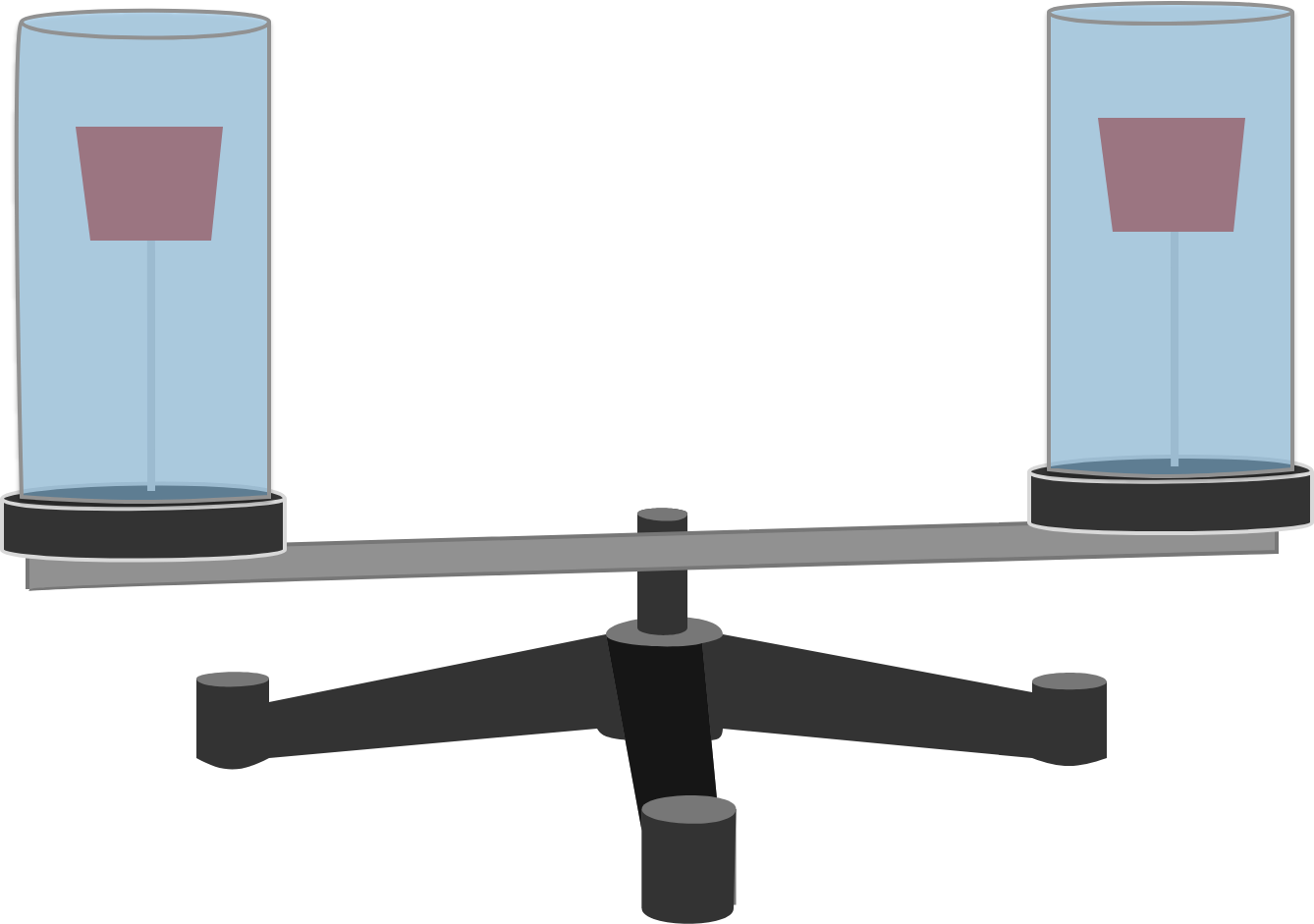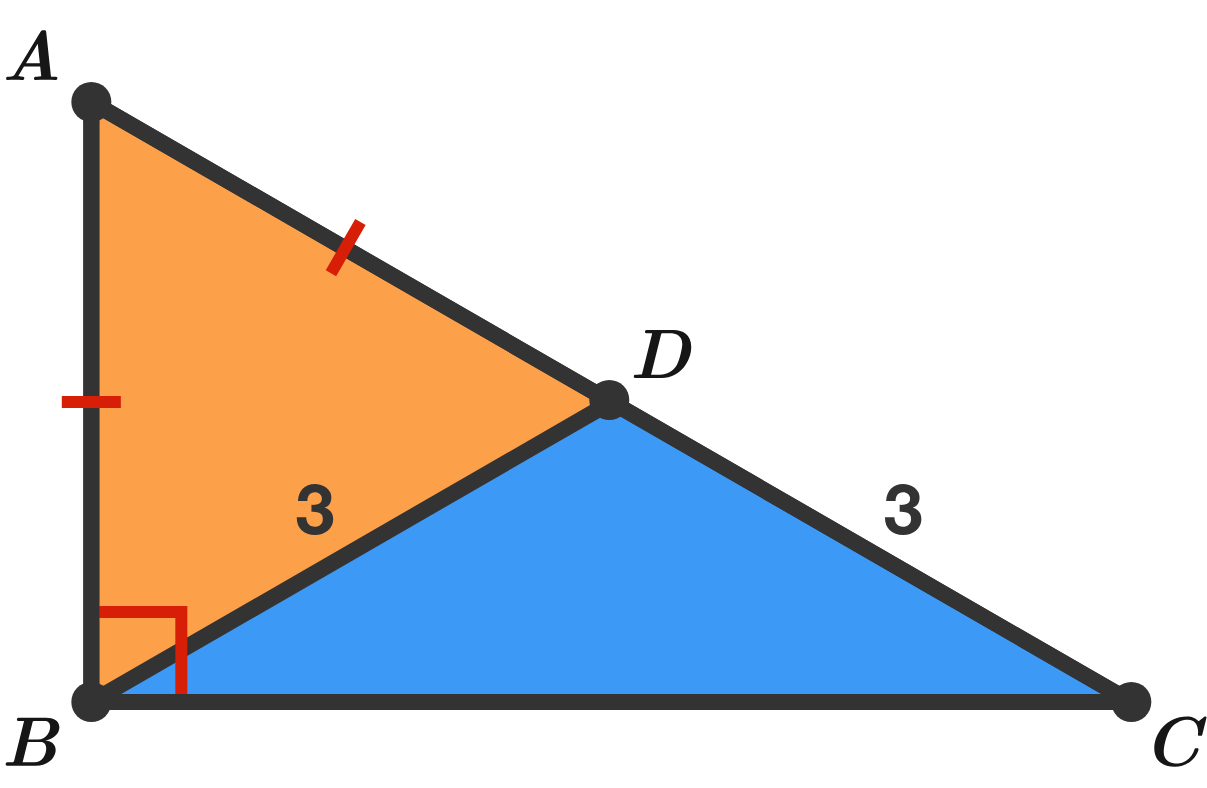# Problems of the Week

Contribute a problem

# 2018-10-08 Basic

$\Huge \color{#69047E}\sqrt{\color{#20A900}3^{\color{#3D99F6}{3}^{\color{#D61F06}{3}}}} \color{#EC7300}=\ ?$

Hint:

• $\displaystyle \large 3^{3^3} = 3^{ \left(3^3 \right) }$
• $\displaystyle \large 3^{3^3} \ne { \left(3^3 \right) }^3$

$\large x = \underbrace{11111...11111}_{\text{Number of 1s = 100}} - \underbrace{22222...22222}_{\text{Number of 2s = 50}}$

What is the sum of digits in $x?$

Hint: Try it with smaller numbers first. For example, find the sum of digits in $111111 - 222.$

I know that just adding numerators and denominators doesn’t work. For example,

$\frac{1}{2}+\frac{2}{3} \ne \frac{1+2}{2+3}.$

Curious if this kind of addition ever works, I look for positive integers $a,b,c,d$ such that

$\dfrac ab + \dfrac cd = \dfrac{a+c}{b+d}.$

After some searching, I conclude that such numbers don't exist. Am I right?A system of two symmetrical, identical, water-filled jars is fixed on a turntable. Each jar has a cork—attached to the bottom by a thread—floating vertically.

What will happen to the corks when the turntable starts rotating?

Details and Assumptions:

• The jars are closed so that no water spills out.

What is the length of $BC?$×